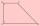Diagonal - 8th grade (13y) - examples

1. Ratio of sidesCalculate the area of a circle that has the same circumference as the circumference of the rectangle inscribed with a circle with a radius of r 9 cm so that its sides are in ratio 2 to 7.
2. Prism 4 sidesThe prism has a square base with a side length of 3 cm. The diagonal of the sidewall of the prism/BG/is 5 cm. Calculate the surface of this prism in cm square and the volume in liters
3. RectangleThe rectangle is 11 cm long and 45 cm wide. Determine the radius of the circle circumscribing rectangle.
4. RhombusCalculate the perimeter and area of ​​a rhombus whose diagonals are 38 cm and 55 cm long.
5. LogsTrunk diameter is 52 cm. Is it possible to inscribe a square prism with side 36 cm?
6. Rhombus ABCDRhombus ABCD, |AC| = 90 cm, |BD| = 49 cm. Calculate the perimeter of the rhombus ABCD.
7. DiagonalCalculate the length of the diagonal of the rectangle ABCD with sides a = 8 cm, b = 7 cm.
8. Square diagonalCalculate length of the square diagonal if the perimeter is 476 cm.
9. IS trapezoidCalculate the length of diagonal u and height v of isosceles trapezoid ABCD, whose bases have lengths a = |AB| = 37 cm, c = |CD| = 29 cm and legs b = d = |BC| = |AD| = 28 cm.
10. Cuboid diagonalCalculate the volume and surface area of the cuboid ABCDEFGH, which sides abc has dimensions in the ratio of 9:3:8 and if you know that the wall diagonal AC is 86 cm and angle between AC and the body diagonal AG is 25 degrees.
11. RhombusIt is given a rhombus of side length a = 29 cm. Touch points of inscribed circle divided his sides into sections a1 = 14 cm and a2 = 15 cm. Calculate the radius r of the circle and the length of the diagonals of the rhombus.
12. RectangleCalculate the length of the side GN and diagonal QN of rectangle QGNH when given: |HN| = 25 cm and angle ∠ QGH = 28 degrees.
13. Rhombus and inscribed circleIt is given a rhombus with side a = 6 cm and the radius of the inscribed circle r = 2 cm. Calculate the length of its two diagonals.
14. RectangleIn rectangle ABCD with sides |AB|=19, |AD|=16 is from point A guided perpendicular to the diagonal BD, which intersects at point P. Determine the ratio ?.
15. RhombusFind the length of the other diagonal and area of rhombus. The perimeter of a rhombus is 40 cm and one of the diagonals is of length 10 cm.
16. Rhombus 2Calculate the area of rhombus which has a height v=48 mm and shorter diagonal u = 60 mm long.
17. Square diagonalCalculate the length of diagonal of the square with side a = 23 cm.
18. PrismCalculate the volume of the rhombic prism. Base of prism is rhombus whose one diagonal is 47 cm and the edge of the base is 28 cm. The edge length of the base of the prism and height is 3:5.
19. Square 2Points D[10,-8] and B[4,5] are opposed vertices of the square ABCD. Calculate area of the square ABCD.
20. TrapezoidAre diagonals in a rectangular trapezoid perpendicular and bisect the angles?

Do you have an interesting mathematical example that you can't solve it? Enter it, and we can try to solve it.

To this e-mail address, we will reply solution; solved examples are also published here. Please enter e-mail correctly and check whether you don't have a full mailbox.